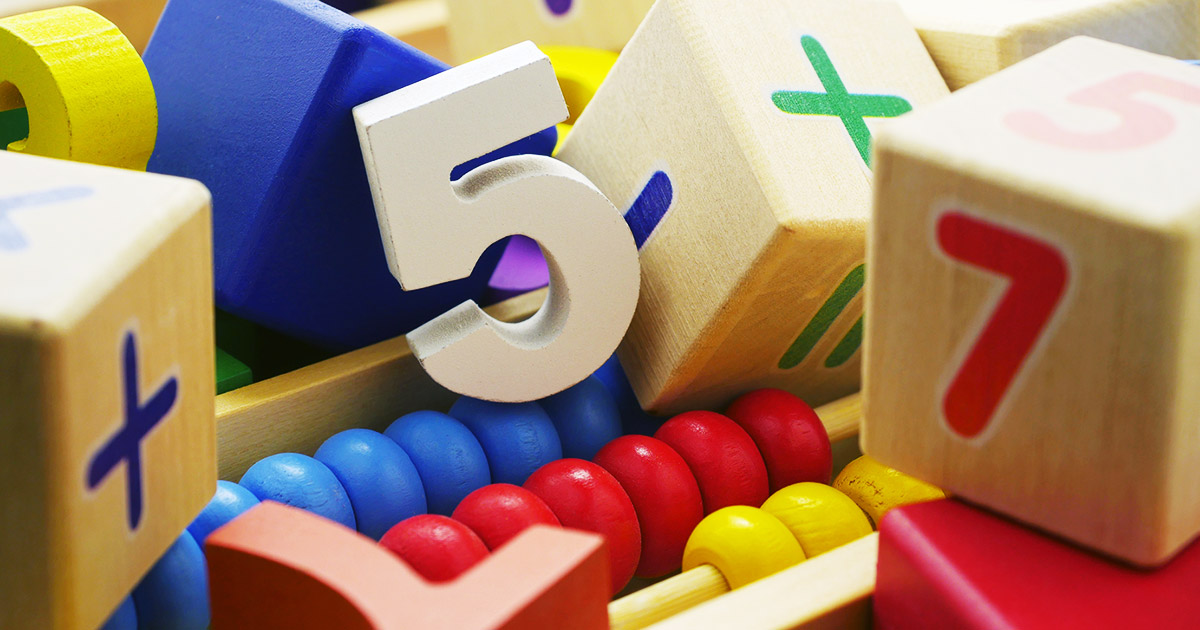# What is Number Sense? The Key to Improve Math Skills

March 20th, 2019

Number Sense is your sense of what numbers mean. What value does a number represent? Which number is bigger or smaller? Making comparisons is also part of this sense. Gersten and Chard say number sense “refers to a child’s fluidity and flexibility with numbers, the sense of what numbers mean and an ability to perform mental mathematics and to look at the world and make comparisons.

Students that struggle with math typically don’t have a good sense of number sense. This is one of the foundational concepts that holds them back from becoming fluid in math. You might think of number sense concepts in terms of the importance phonemic awareness plays in reading. Number sense plays that same role in math.

In the past, teachers have concentrated on math facts and how to do calculations. Now, after years of research, they have found that the reason students struggle with learning math is because they don’t have the underlying foundation of what numbers actually mean: number sense. Common core is addressing this. Most new textbooks are addressing this. However, if you don’t have that basic foundational skill, the new way of teaching math will still be difficult. We need to spend more time on mastering number sense.

### An Example of Number Sense

I have a student in Australia that needed to add 6 plus 5. He couldn’t start at the number 6 and say 6 and then count up 5 more. He needed to count 1, 2,3, 4, 5, 6, and then 7, 8, 9, 10, 11. He does not have a strong sense of ‘number sense.’

## Watch the First Number Sense Lesson

Number sense incorporates counting, proportional thinking, as well as whole and parts.

### Counting

Counting involves counting by ones, twos, fives, tens, and more. Counting is the ability to put names to quantities. It is understanding how our number system is organized in groups of 10 – base 10. Most students that struggle with math lack counting skills. Number sequence, skip-counting, and patterns (odd and even) are difficult for them.

### Counting Activities

Counting involves counting by ones, twos, fives, tens, and more. Most students that struggle with math lack counting skills. Number sequence, skip-counting, and patterns (odd and even) are difficult for them.

Count up to 20 and down from 20.

Count by 10’s to 100. Count-down from 100 by 10’s.

Start at 22 and count up by 10’s to 102.

Start at 86 and count down by 10’s.

### Proportion

Proportions in math deals with which is more and which is less. Comparing quantities and proportions to each other such as a slice of pizza from a small pizza, medium size pizza, and a large pizza. Proportional thinking is thinking about how many times bigger or times smaller a number is compared to another number (e.g. How many times bigger is 6 than 3? How many times smaller is 3 than 6? How many times bigger is your mom’s arm than your arm?

### Proportional Activities

1. Measure how tall you are with a string. Cut the string and use it to discover what else is as big as you in the classroom or at home.
2. Categorize items that are bigger than they are and smaller than they are.
3. Look for items that are twice your size.
4. Look for items that are half your size.

Another example:

• If you have 8 pieces of pizza to divide between 4 friends, how many pieces of pizza should each friend get?
• Six workers can build a house in 3 days. Assuming that all of the workers work at the same rate, how many workers
would it take to build a house in 1 day?

### Wholes and Parts

This is understanding parts of a number. In other words, number families. For example, understanding that 8 is made up of  7 and 1, 6 and 2, 5 and 3, as well as 4 and 4. Another example is 7 plus 3 = 10. The parts are 7 and 3; the whole is 10. “The whole is equal to the sum of its parts.” (Euclid). Fractions are examples of wholes and parts. 1/4 + 3/4 = 4/4 = 1 whole.

### Wholes and Parts Activities

This is where you start to learn about and master fractions. Fractions are equal parts of a whole.

Which pictures are showing fractions?

Answer to Wholes and Parts Activity

Remember: Fractions always need to show equal parts. The correct answers are figures 1 – showing halves and figure 4 – showing thirds.

Figure 2 shows 4 the whole divided into 4 random parts (all 4 parts would need to be equal in order to be an example of fractions).

Figure 3 shows the whole circle divided into 3 random sizes (all 3 parts would need to be equal sizes to be an example of fractions).

For more direct instruction on improving math skills with number sense lessons, check out our Awaken the Scholar Within Math Program. Here, Bonnie teaches these skills to you, step-by-step through audio and video lessons. The program includes the BT Easy Math Reference Guide and Super Spacers which integrates both number sense and traditional math skills seamlessly.

Bonnie Terry is a Board Certified Educational Therapist and internationally recognized as America’s Leading Learning Specialist and the founder of BonnieTerryLearning.com. Terry is an expert in developing learning programs that target how people learn through the visual, auditory, and tactile/kinesthetic processing systems. Terry coaches teachers and parents so they can give their child a 2 to 4-year learning advantage in just 45-60 minutes a day. She is a frequent media guest and speaker.

### Blog Topics